# Correlation Pearson Product Moment Using SPSS

Correlation Pearson Product Moment Using SPSS | Correlation test used to determine the level of the relationship between the study variables. Pearson Product Moment Correlation suitable for research data in the form of a ratio. Pearson Product Moment correlation test can produce a correlation coefficient that shows: the relationship, the degree of relationship, and the direction of the relationship (positive or negative).

Decision-making process in the Correlation
To see whether there is a relationship between variables can be guided by:
1. If the Sig. <0.05, then there is a significant relationship between the variables of the study.
2. If the Sig. > 0.05, then there is no significant relationship between the variables of the study.

Directions correlations ranged from 0.00 to 1.00 + are a positive sign and the sign - is negative. The degree of relationship between variables can be based on the value of Pearson Correlation:
1. Value Pearson Correlation 0.00 to 0.20, meaning almost no correlation.
2. Value Pearson Correlation 0.21 to 0.40, meaning a low correlation.
3. Value Pearson Correlation 0.41 to 0.60, meaning that the correlation being.
4. Value Pearson Correlation 0.61 to 0.80, meaning that a high correlation.
5. Value Pearson Correlation 0.81 to 1.00, meaning that perfect correlation.

Case in Correlation

A company manager wants to know whether there is a relationship between competences significant with Employee Performance. For the manager of the collected data Competence and Performance of 40 samples of employees. The research data as shown below.Step By Step to Correlation Using SPSS

1. Turn on the SPSS program and select the Variable View, furthermore, in the Name write Competency and Performance. Ignore the other options...2. The next step, click the Data View and enter the data Competency and Performance.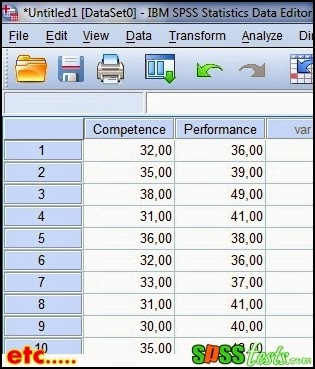3. Next, from the SPSS menu click Analyze - Correlate - Bivariate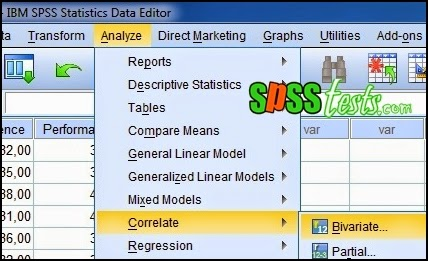4. It will appear Bivariate Correlations dialog box, then enter Competence and Performance Variables box, on the Correlations Coefficients select Pearson , on the Test of Significance select Two-tailed, then check Flag significant correlations5. The last step clicks Ok, after which it will appear SPSS output, as follows:Interpretation of Results Output Correlation

Based Output Correlations obtained sig. (2-tailed) of 0.000 <0.05, it can be concluded that there is a significant relationship between competence with Employee Performance. In addition, Pearson correlation values obtained for 0,692, so it can be said that the competence correlated with high employee performance.

Next: Step by Step Simple Linear Regression Analysis Using SPSS

[Keywords: Pearson Product Moment Correlation Using SPSS | How to Conduct Pearson Product Moment Correlation Test in SPSS | Step By Step Perform Pearson Product Moment Correlation Using SPSS | Tutorial Pearson Product Moment Correlation with SPSS Software Complete]
[Image: Data SPSS Version 21]
[Source: Summarized from various sources]

### 6 Responses to "Correlation Pearson Product Moment Using SPSS"

1.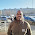Nice presentation, very helpful

1.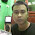2.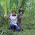I have a question can I ask here.

1.3.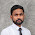it's really helpful. Thank you

4.Very Helpful , I Request you to add more examples of SPSS tests